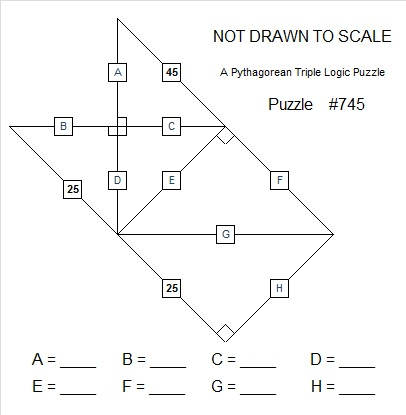# 745 Pythagorean Triple Puzzle

• 745 is a composite number.
• Prime factorization: 745 = 5 x 149
• The exponents in the prime factorization are 1 and 1. Adding one to each and multiplying we get (1 + 1)(1 + 1) = 2 x 2 = 4. Therefore 745 has exactly 4 factors.
• Factors of 745: 1, 5, 149, 745
• Factor pairs: 745 = 1 x 745 or 5 x 149
• 745 has no square factors that allow its square root to be simplified. √745 ≈ 27.294688.PUZZLE DIRECTIONS: This puzzle is NOT drawn to scale. Angles that are marked as right angles are 90 degrees, but any angle that looks like a 45 degree angle, isn’t 45 degrees. Lines that look parallel are NOT parallel. Shorter looking line segments may actually be longer than longer looking line segments. Most rules of geometry do not apply here: in fact non-adjacent triangles in the drawing might actually overlap.

No geometry is needed to solve this puzzle. All that is needed is the table of Pythagorean triples under the puzzle. The puzzle only uses triples in which each leg and each hypotenuse is less than 100 units long. The puzzle has only one solution.

Today’s Pythagorean triple puzzle has only 2 more triangles than last week’s puzzle, but it shouldn’t be any more difficult. Please, give it a try!Print the puzzles or type the solution on this excel file: 10 Factors 2016-01-18————————————-

Here are some fun facts about the number 745:

745 can be written as the sum of consecutive numbers three different ways:

• 372 + 373 = 745; that’s 2 consecutive numbers
• 147 + 148 + 149 + 150 + 151 = 745; that’s 5 consecutive numbers
• 70 + 71 + 72 + 73 + 74 + 75 + 76 + 77 + 78 + 79 = 745; that’s 10 consecutive numbers

745 can also be written as the sum of the thirteen prime numbers from 31 to 83. See if you can name all those primes while you add them up.

745 is the sum of two squares two different ways:

• 27² + 4² = 745
• 24² + 13² = 745

Both of 745’s prime factors are hypotenuses of Pythagorean triples, so 745 is the hypotenuse of FOUR Pythagorean triples:

• 216-713-745; calculated from 2(27)(4), 27² – 4², 27² + 4².
• 255-700-745
• 407-624-745; calculated from 2(24)(13), 24² – 13², 24² + 13².
• 447-596-745

5 is the greatest common factor of one of the non-primitive triples while 149 is the greatest common factor of the other. Which is which?

If you check any of those triples, you will see that 745² is 555025, which is a cool looking number, too.

745 is also the sum of three squares three different ways:

• 18² + 15² + 14² = 745
• 22² + 15² + 6² = 745
• 24² + 12² + 5² = 745

745 is a palindrome in three different bases, two of which are consecutive:

• 454 BASE 13; note that 4(13²) + 5(13) + 4(1) = 745.
• 3B3 BASE 14 (B= 11 base 10); note that 3(14²) + 11(14) + 3(1) = 745.
• 171 BASE 24; note that 1(24²) + 7(24) + 1(1) = 745.

————————————-

## 2 thoughts on “745 Pythagorean Triple Puzzle”

1.Paula Beardell Krieg

Great charts! Chart of Pythagorean triplets are not something that comes along ever day.

Why are some squares blue and other yellow in your chart? And then there is some color coding with red and black too. Did you explain this in a post I’ve missed? I tried to sort it out,but this was my last stop before heading to bed….brain just isn’t functioning. thanks.

2.Steve Morris

“Lines that look parallel are NOT parallel.
Any angle that looks like a 45 degree angle, isn’t 45 degrees.”